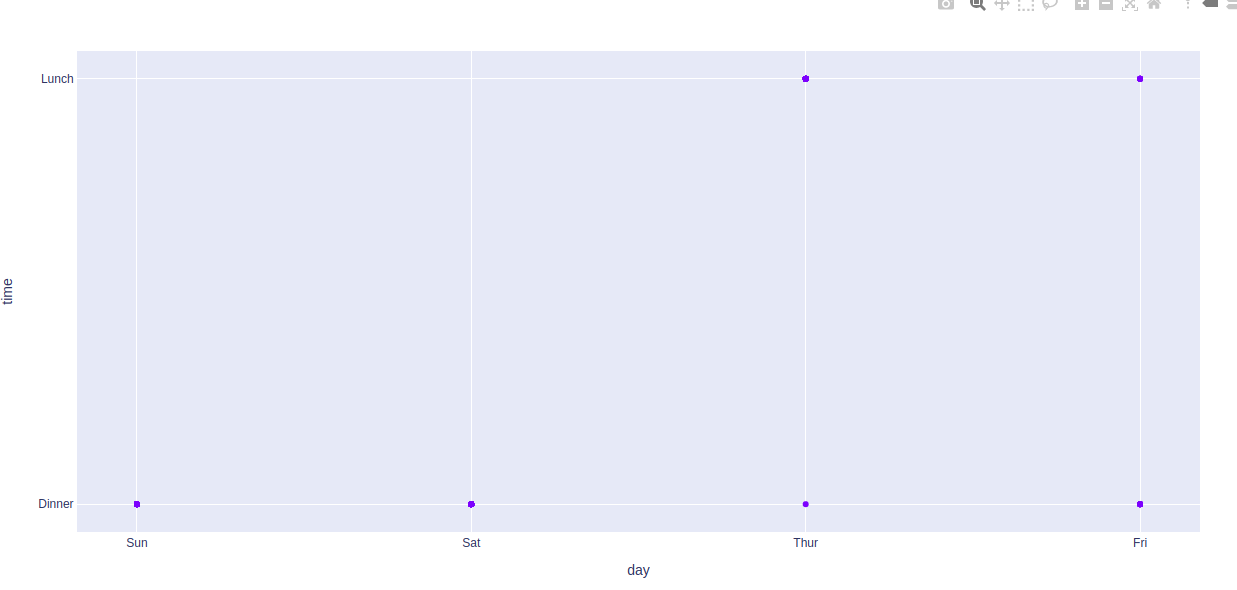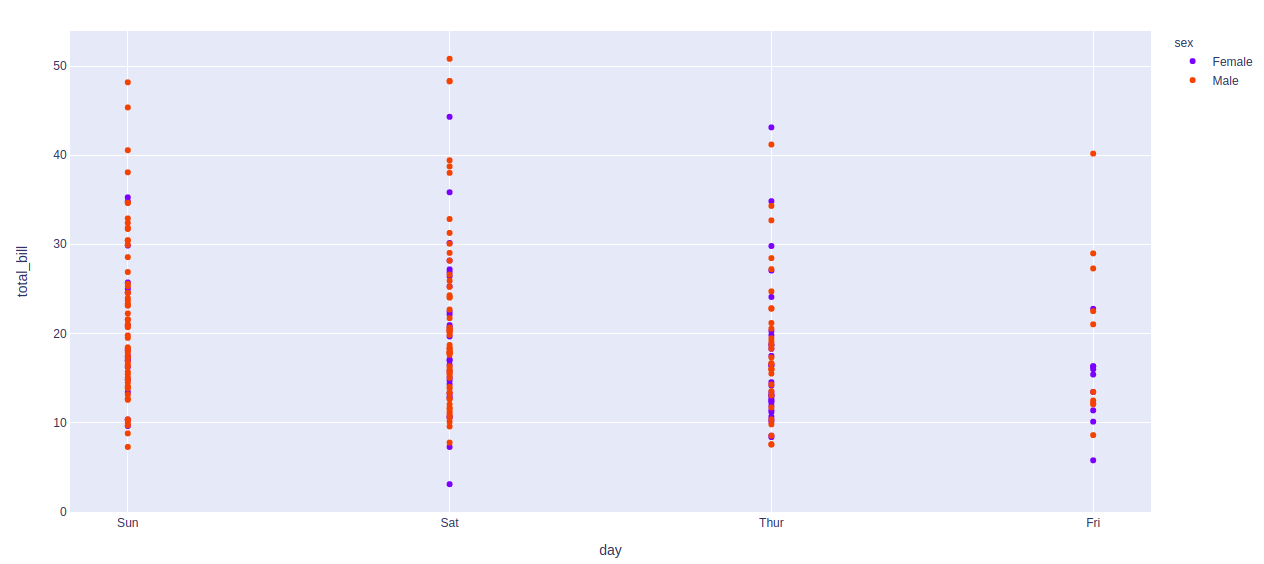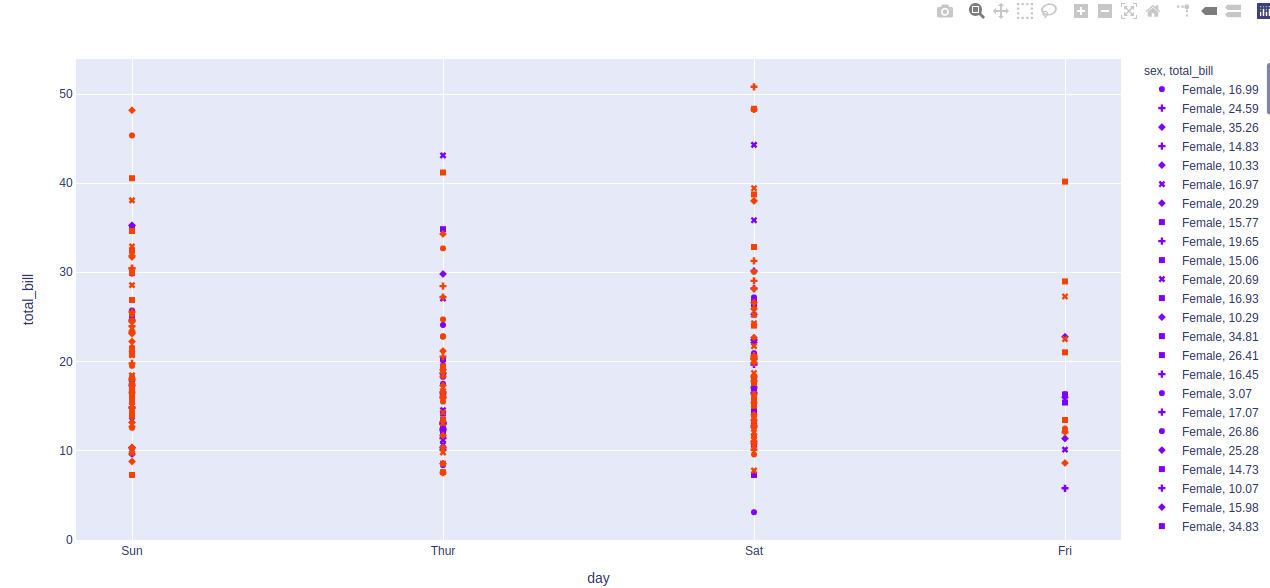# plotly.express.scatter() function in Python

• Last Updated : 17 Jul, 2020

Plotly library of Python can be very useful for data visualization and understanding the data simply and easily. Plotly graph objects are a high-level interface to plotly which are easy to use.

## plotly.express.scatter() function

This function is used to create the scatter plot and can be used with pandas dataframes. Each row of dataframe is represented by a symbol mark in 2D space in scatter plot.

Syntax: plotly.express.scatter(data_frame=None, x=None, y=None, color=None, symbol=None, size=None, hover_name=None, hover_data=None, title=None, template=None, width=None, height=None)

Parameters:

data_frame: DataFrame or array-like or dict needs to be passed for column names.

x, y: This parameters is either a name of a column in data_frame, or a pandas Series or array_like object. Values from this column or array_like are used to position marks along the x and y axis in cartesian coordinates respectively.

color: This parameters assign color to marks.

symbol: This parameter is used to assign symbols to marks. It is either a name of a column in data_frame, or a pandas Series or array_like object.

size: This parameter is used to assign mark sizes. It is either a name of a column in data_frame, or a pandas Series or array_like object.

hover_name:  Values from this column or array_like appear in bold in the hover tooltip.

hover_data: This parameter is used to appear in the hover tooltip or tuples with a bool or formatting string as first element, and list-like data to appear in hover as second element Values from these columns appear as extra data in the hover tooltip.

custom_data: This parameter is either names of columns in data_frame, or pandas Series, or array_like objects

Example 1:

## Python3

 `# Python program to demonstrate scatter``# plot`` ` `import` `plotly.express as px`` ` `df ``=` `px.data.tips()`` ` `plot ``=` `px.scatter(df, x ``=` `'day'``, y ``=` `'time'``)``plot.show()`

Output:Example 2: Coloring according to the sex in tips dataset

## Python3

 `# Python program to demonstrate scatter``# plot`` ` `import` `plotly.express as px`` ` `df ``=` `px.data.tips()`` ` `plot ``=` `px.scatter(df, x ``=` `'day'``,``                  ``y ``=` `'total_bill'``, ``                  ``color``=``'sex'``)``plot.show()`

Output:Example 3: Using the symbol parameter

## Python3

 `# Python program to demonstrate scatter``# plot`` ` `import` `plotly.express as px`` ` `df ``=` `px.data.tips()`` ` `plot ``=` `px.scatter(df, x ``=` `'day'``, ``                  ``y ``=` `'total_bill'``,``                  ``color``=``'sex'``, ``                  ``symbol ``=` `'total_bill'``)``plot.show()`

Output:My Personal Notes arrow_drop_up